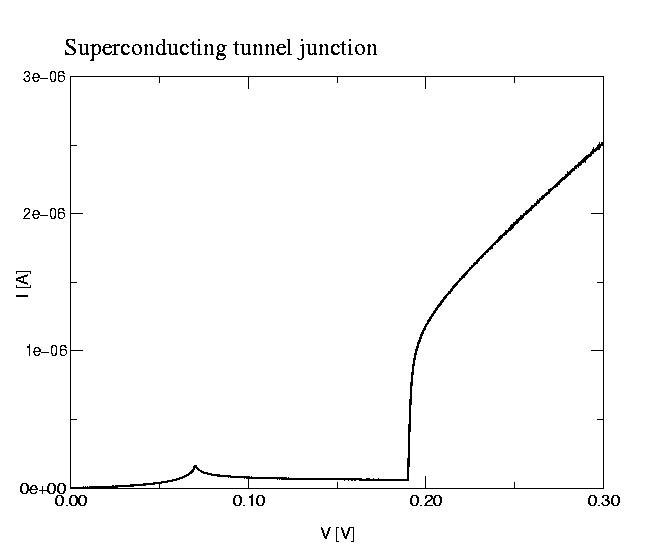# Example simulations with SIMON

On this page we show interesting examples of single-electron circuits which have been proposed and sometimes even built and characterized. We compare measurement with simulation to show the capabilities of SIMON and to give you a better understanding of this analysis software. This page should help you answer the question, if SIMON could help you in your work.
However, each example is interesting on its own, regardless of if you need a single-electron simulator.

## Cotunneling Comparison: Theory - SIMON

Averin and Nazarov give in "Single Charge Tunneling - Coulomb Blockade Phenomena", 1992 on page 223 an analytic approximation to the current through a double tunnel junction including cotunneling. Their equation (16) is valid for small bias voltages and low temperatures. We are comparing here this equation to the simulation result achieved with SIMON. The circuit is a simple doubel junction (no gate capacitor) with both tunnel resistances being 100k and both capacitances being 1aF. Two simulations at 0K and 20K have been performed. As one can clearly see, SIMON's result agrees very well with the analytic approximation.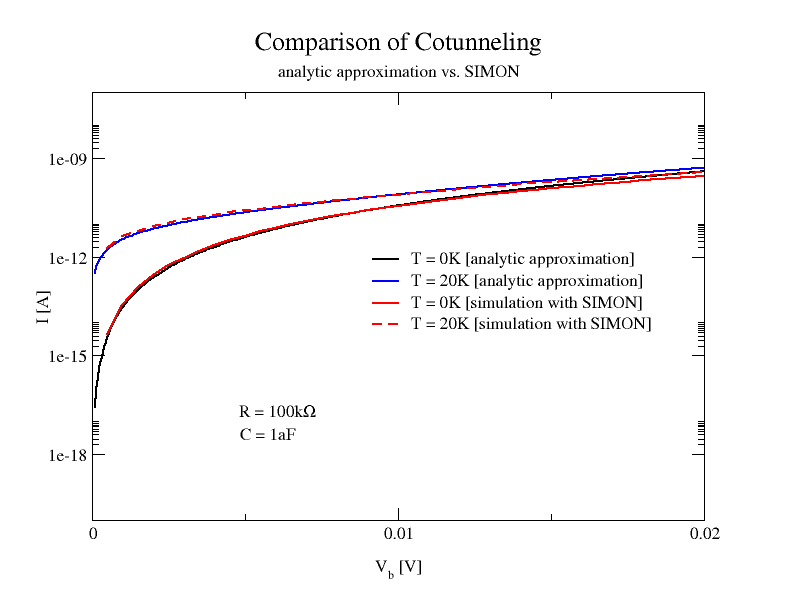## Tunneling Phase Logic Memory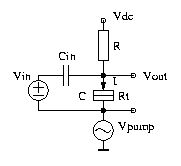The tunneling phase logic (TPL) is an idea of Richard Kiehl (see for example the Journal of Applied Physics article 'Operation of bistable phase-locked single-electron tunneling logic elements' by T. Ohshima and R. Kiehl, 80 (2), 1996, p. 912-923). The principle is to use Coulomb oscillations of a single current biased tunnel junction. These oscillations can lock to a pump in a bistable fashion. The input signal determines to which phase the oscillations lock. In the graphs below one can see the memory operation. On the very bottom in gray one can see the two possible phase locked Vout signals (solid and broken line). We first make Vin equal to zero with switched on Vdc. The oscillations lock to the solid-line phase. Then we switch Vin from low to high. The phase-lock is not altered. Only if Vdc is cycled to low and high again can the phase lock to the second phase (broken-line). This means the circuit once phase-locked retains its phase-lock independent of the input signal. This is very usefull for a memory cell. The simulation shows that SIMON can handle normal resistors as well as time dependent bias signals.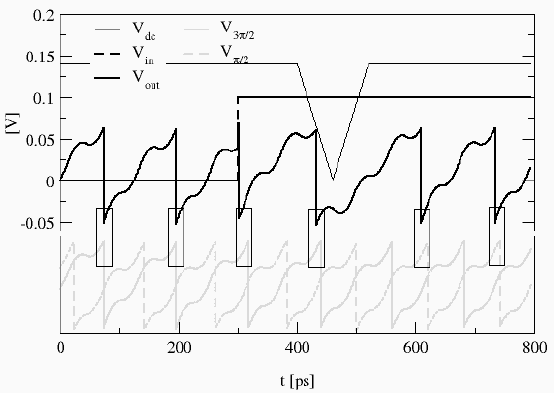## SET with discrete energy levels - artificial atom: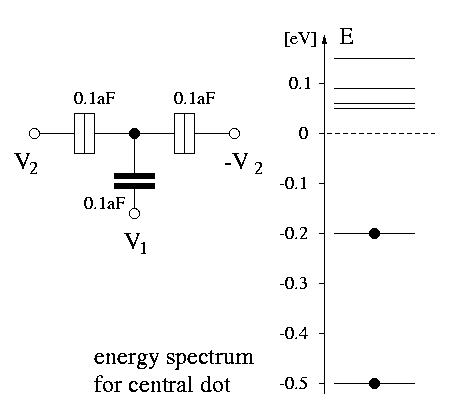Today it is possible to manufacture SETs where the quantum mechanical confinement energy is large enough that one can observe effects of single energy levels. On the left side is shown a quantum dot with discrete energy spectrum. In this case the Coulomb blockade mixes with the discrete energy spectrum and produces a rich signature. The stability plot is an ideal visualization of such behavior.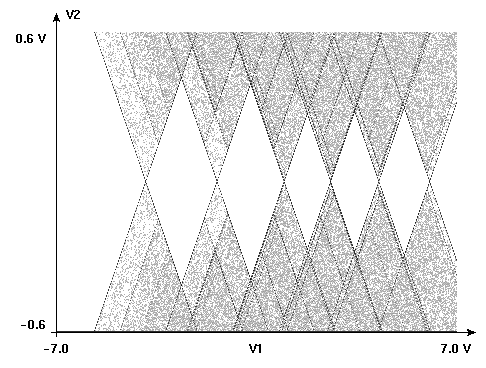The picture on the left shows a stability plot simulated with SIMON. It shows the dI/dV2, where 2*V2 is the bias voltage and I the current through the SET. Different sized diamonds and lines running parallel to diamond edges are clearly visible. You can qualitatively compare this plot to the measurement published in the article "Excitation Spectra of Circular, Few-Electron Quantum Dots" by Kouwenhoven et al. in Science, Vol 278, December 1997. The image is reproduced with permission below. Qualitatively the simulation produces the same result. The particular energy levels were not known to me so I couldn't simulate the exact same device.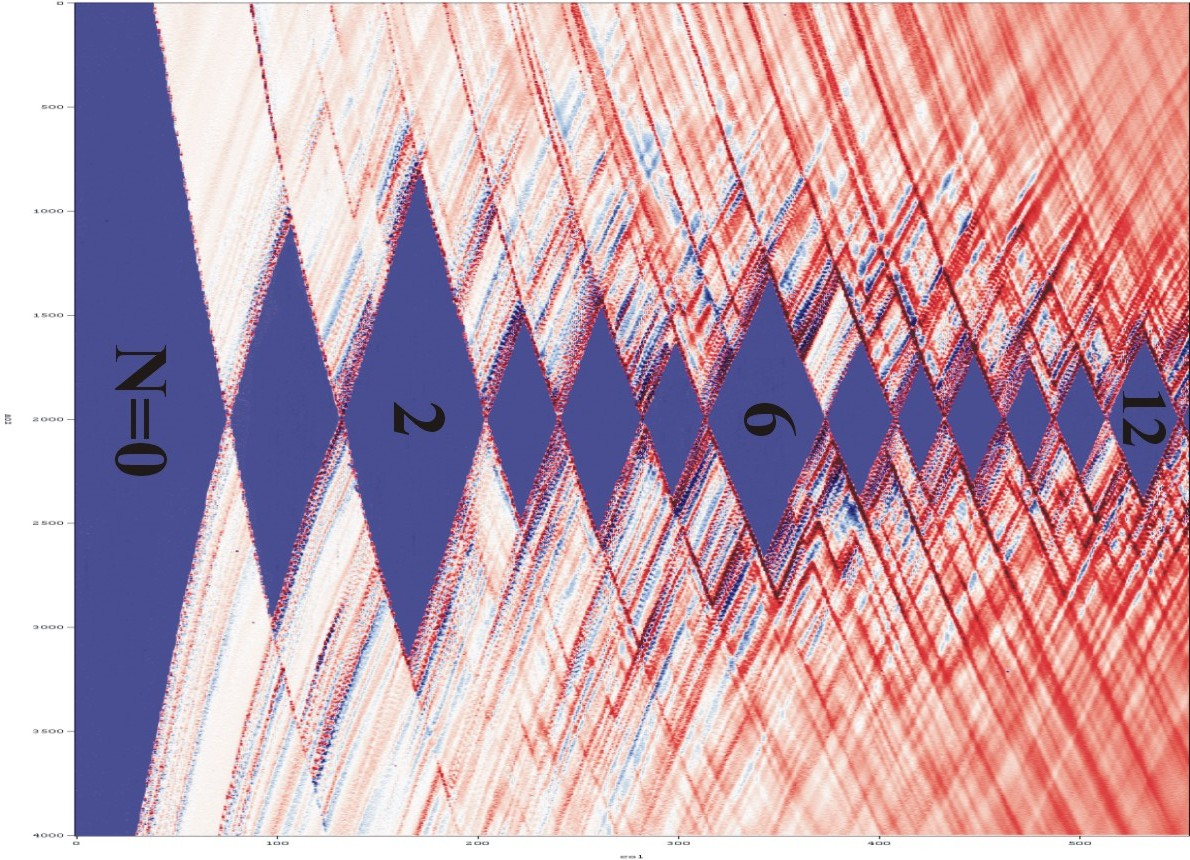## Negative-Differential-Resistance Circuit: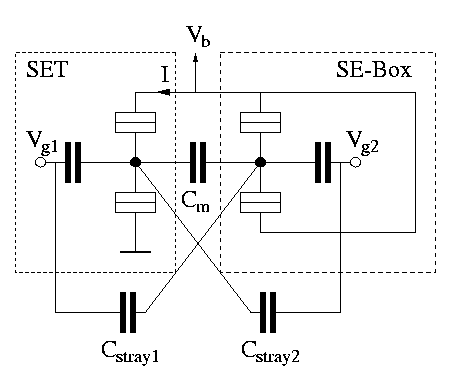This circuit was originally an idea of Paul McEuen and David Dixon and was later published by Heij, Dixon, Hadley, and Mooij . This circuit works in the following way. The SET and the SE-Box have a certain threshold voltage. For the SET it is the threshold to conduct a current I. For the SE-Box it is the threshold to admit one more electron into the box. If the threshold of the SET is lower than the threshold of the SE-Box the circuit may exhibit a NDR in the following way. Assume the bias voltage Vb is slowly raised from zero. At first SET and SE-Box are in Coulomb blockade. At a certain point the SET will start to conduct current which increases with increasing bias voltage. When the bias voltage reaches the threshold of the SE-Box an electron will tunnel onto the central island of the box. The coupling capacitance Cm makes sure that the SET is influenced by the electron entering the box. This influence can be in such a manner, that the SET is put back into Coulomb blockade. This means that the current will decrease or even go back to zero, resulting in a negative-differential-resistance. Increasing the bias voltage Vb even more will finally make the SET conduct current despite electrons entering or exiting the box. Thresholds of SET and SE-Box can be tuned by Vg1 and Vg2 respectively. This device was built, measured, and simulated by the same authors Heij et. al. The measured stability plot is reproduced here with their permission.Black regions show NDR onset. A very similar stability plot can be simulated with SIMON. The simulation shows very good agreement with the measurement.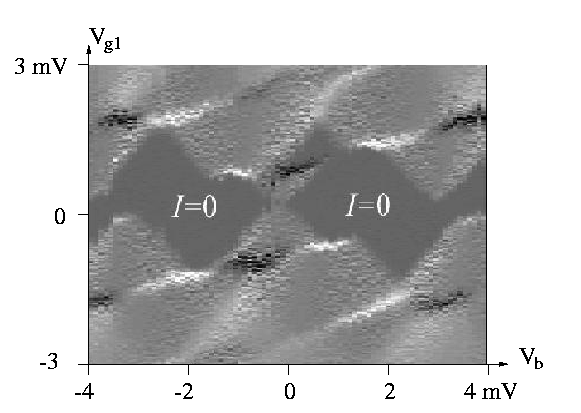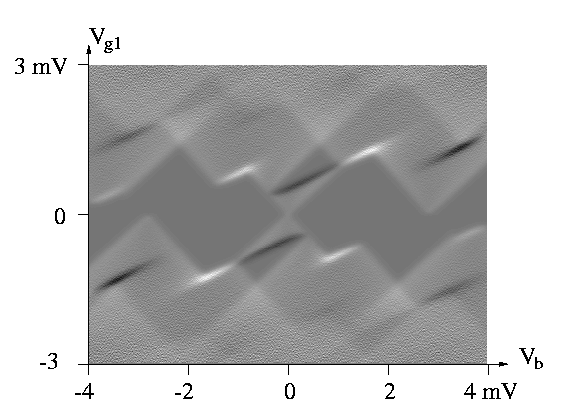## Pump

A single-electron pump consisting of three tunnel junctions and the characteristic 'bee hive' stability plots are shown for three different temperatures 0K, 30K and 100K.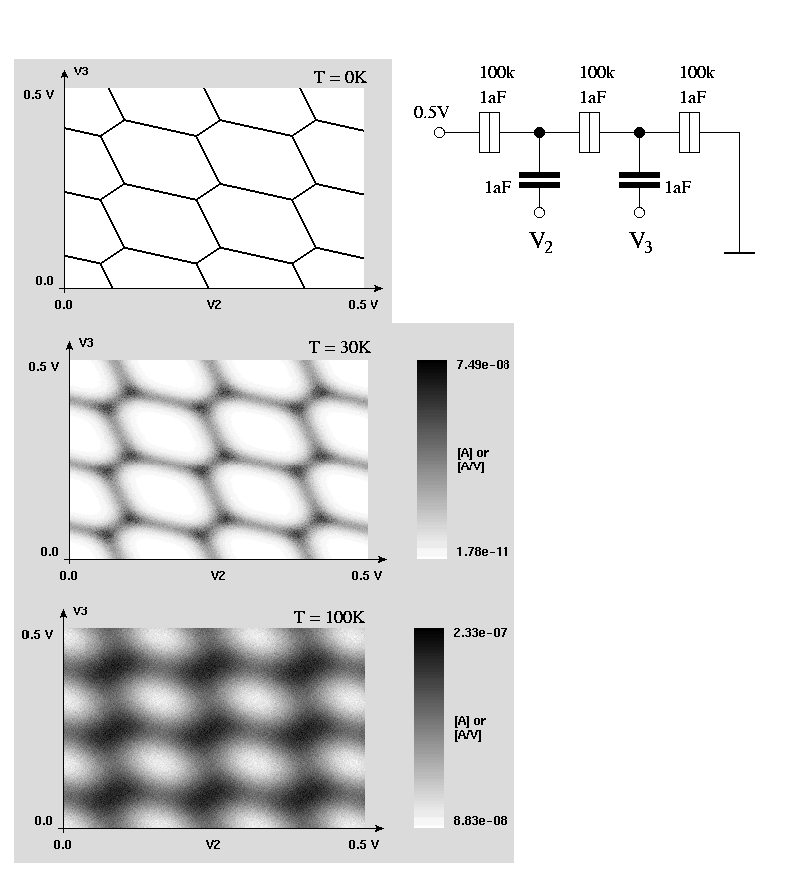## Esaki tunnel diode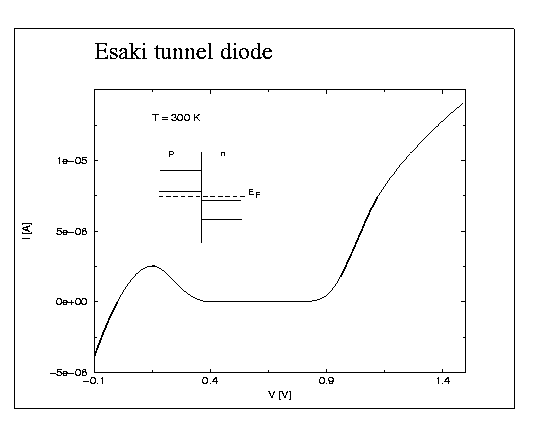## Superconducting tunnel junction

The theory behind this plot can be found in "Introduction to Superconductivity" by Tinkham.